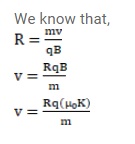# Consider the situation of the previous problem.

Question:

Consider the situation of the previous problem. A particle having charge $\mathrm{q}$ and mass $\mathrm{m}$ is projected from the point $Q$ in a direction going into the plane of the diagram. It is found to be describe a circle of radius $r$ between the two plates. Find the speed of the charged particle.

Solution: